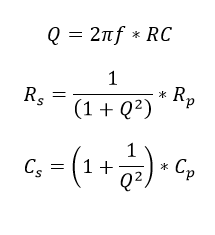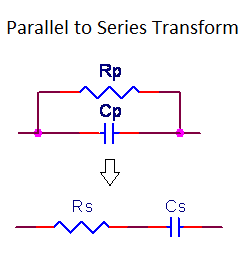# RC Paralel to Series Impedance Transform Calculator

This CalcTown calculator calculates the equivalent resistance and capacitance values in series for a given parallel combination of the same.

Serial/parallel R-C networks are ubiquitous in low frequency and RF designs.  The necessity to view these simple circuits in both serial and parallel forms often recurs, especially during analysis.

#### Result

Ω
FWhere,

Rs = Resistance in series

Rp = Resistance in parallel

f = Operating Frequency

Q = Quality factor

Cs = Capacitance in series

Cp = Capacitance in parallel